PythonTip 里未攻克的题目，如取石子游戏，如今积累工作经验之后从新挑战，成功了。把过程记录分享下。

### 分析（动态规划）：¶

(x,y)的结果显然决定与前面已填好的(m,n) , 其中m,n 分别小于 x,y 。 所以可以用动态规划。 根据之前失败坐标的集合推算本行的失败坐标。

#### 错误填表分析过程¶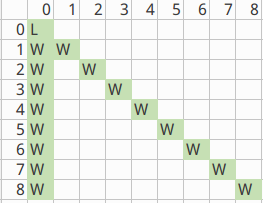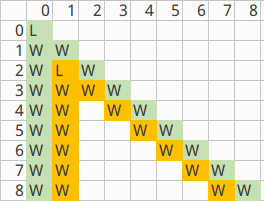#### 正确填表分析过程¶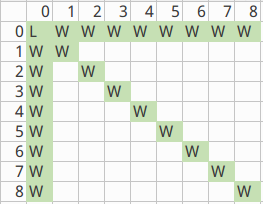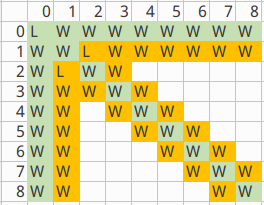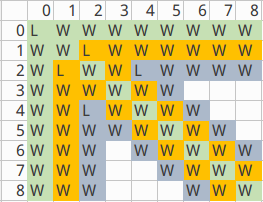### Python代码:¶

```################################################################################
# print "T: 二维坐标表示法, 每行至多有一个失败坐标。二维表只填半边导致失误，改正"
################################################################################
if a < b: a,b = b,a

m = [(0,0)]  # 失败的坐标纪录池
for i in range(1,a+1):
for j in range(i+1):
# if i == j or j == 0: # win
#     continue

for x in m:
if (i-x) == (j-x) or i == x or j == x: # win
break
else:  # else 是for的部分，break for的时候也break了else
m.append((i,j)) # 版本一：二维表只填半边，所以只有这行代码
m.append((j,i)) # 版本二：二维表两边都填，多加这一行就OK了
#print i,j,m

print "Loose" if (a,b) in m else "Win"
```